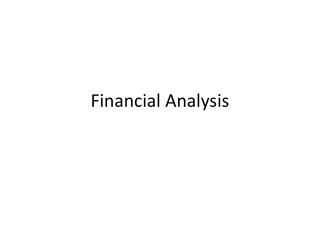# Financial Analysis - PowerPoint PPT PresentationDownload PresentationFinancial Analysis

Financial AnalysisDownload Presentation## Financial Analysis

- - - - - - - - - - - - - - - - - - - - - - - - - - - E N D - - - - - - - - - - - - - - - - - - - - - - - - - - -
##### Presentation Transcript

1. Financial Analysis

2. Quantitative Analysis • Systematic analysis of key elements based on analysis context • Quantitative techniques to standardize financial information for relevant comparisons • In-depth analysis for key factors, including “red flags”

3. Useful Financial Comparisons • Benchmarks: rules of thumb or averages • Trend Analysis (analysis over time) • Industry Averages • Market Averages

4. Common-size Analysis • Overview vs. detail • Balance sheet: total assets = 100% • Income Statement: sales (or total revenues) = 100% • Comparisons over time & across firms (or industry averages) • Useful starting point for financial overview

5. Ratio Analysis • A ratio converts financial information to a percentage, one approach to standardization • Each ratios provides a somewhat different analysis • Ratios overlap—a problem in one area should show up as problems in other areas • The importance of specific ratios differs, based on the purpose of the financial analysis • Ratios for the most recent period are usually the most important

6. Ratio Categories • Liquidity—cash, working capital & cash flow related • Leverage—debt & solvency analysis • Activity—turnover ratios as possible efficiency measures • Performance (or profitability)—bottom line or earnings related • Market ratios—measuring stock performance relative to financial information

7. Liquidity Ratios • Current ratio: current assets/current liabilities • Cash ratio: (cash + marketable securities)/current liabilities

8. Leverage Ratios • Debt to equity ratio: total liabilities/total stockholders’ equity • Debt to market equity: total liabilities at book value/total equity at market value

9. Activity Ratios • Inventory turnover: cost of sales/average inventory • Receivables turnover: sales/average accounts receivable

10. Activity Ratios in Days • Average days inventory in stock: 365/inventory turnover • Average days receivables outstanding: 365/receivables turnover • Length of operating cycle: average days inventory + average days receivables

11. Profitability • Return on sales: net income/sales • Return on total equity: net income/average stockholders’ equity

12. Market-based Ratios • Price earnings ratio (PE): Stock price / EPS • Dividend Yield: Dividend per share / Stock price • Price Earnings to Growth (PEG) = PE based on forecast EPS / 5-year earnings forecast